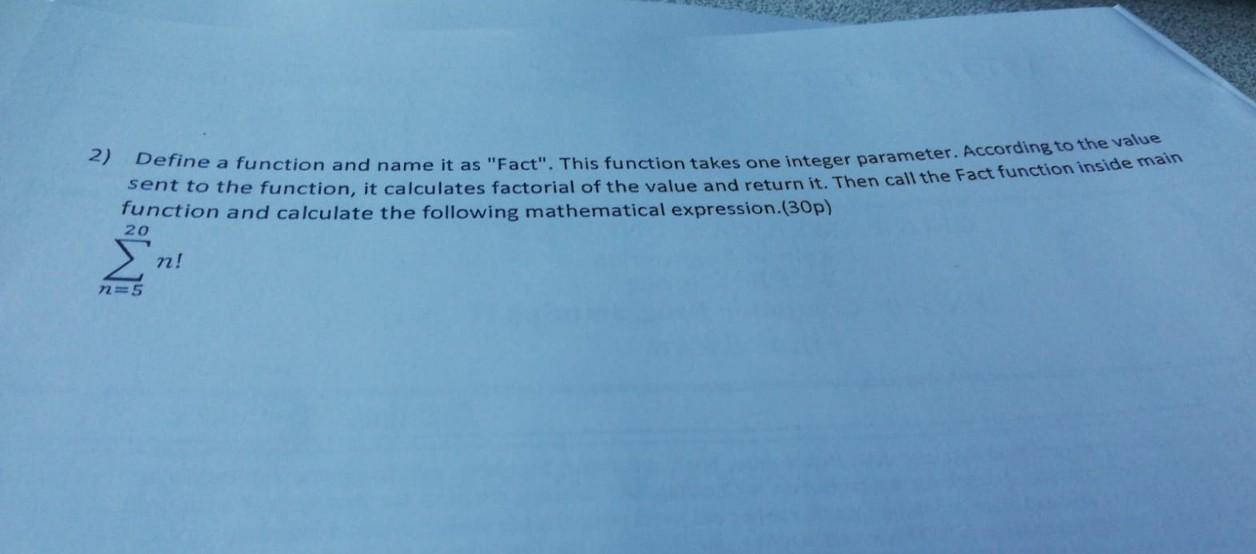# (Solved):   in C programming please 2) Define a function and name it as "Fact". This function takes o ...2) Define a function and name it as "Fact". This function takes one integer parameter. According to the value sent to the function, it calculates factorial of the value and return it. Then call the Fact function inside main function and calculate the following mathematical expression.(30p) $\sum_{n=5}^{20} n !$